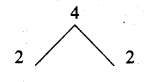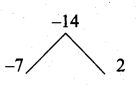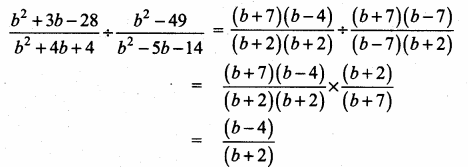## Ex 3.5 | Aster Classes

Tamilnadu, Samacheer, Kalvi, 10th, sslc, Maths, Solutions, Chapter 3, Algebra, Ex 3.5,

Question 1.
Simplify
(i) \frac{4 x^{2} y}{2 z^{2}} \times \frac{6 x z^{3}}{20 y^{4}}

(ii) p2−10p+21p−7×p2+p−12(p−3)2
P2 – 10p + 21 = (p – 7) (p – 3)
p2 + p – 12 = (p + 4) (p – 3)

(iii) 5t34t−8×6t−1210t

Question 2.
Simplify
(i) x+43x+4y×9×2−16y22x2+3x−20
9x2 – 16y2 = (3x)2 – (4y)2
= (3x + 4y) (3x – 4y)
2x2 + 3x – 20 = 2x2 + 8x – 5x – 20
= 2x (x + 4) – 5 (x + 4)
= (x + 4) (2x – 5)

(ii) x3−y33x2+9xy+6y2×x2+2xy+y2x2−y2
x3 – y3 = (x – y) (x2 + xy + y2)
x2 + 2xy + y2 = (x + y) (x + y)

3x2 + 9xy + 6y2 = 3(x2 + 3xy + 2y2)
= 3 (x + 2y) (x + y)
(x2 – y2) = (x + y) (x – y)

Question 3.
Simplify
(i) 2a2+5a+32a2+7a+6÷a2+6a+5−5a2−35a−502 a2 + 5a + 3a + 3 = 2a2 + 2a + 3a + 3
= 2a(a + 1) + 3 (a + 1)
= (a + 1) (2a + 3)2a2 + 7a + 6 = 2a2 + 3a + 4a + 6
= a(2a + 3) + 2 (2a + 3)
= (2a + 3) (a + 2)a2 + 6a + 5 = (a + 5) + (a + 1)
-5a2 – 35a – 50 = -5(a2 + 7a + 10)
= -5(a + 5)(a + 2)

(ii) b2+3b−28b2+4b+4+b2−49b2−5b−14
Solution:

b2 + 3b – 28 = (b + 7) (b – 4)
b2 + 4b + 4 = (b + 2) (b + 2)
b2 – 49 = b2 – 72
= (b + 7) (b – 7)
b2 – 5b – 14 = (b – 7) (b + 2)(iii) x+24y+x2−x−612y2

x2 – x – 6 = (x – 3) (x + 2)

(iv) 12t2−22t+83t÷3t2+2t−82t2+4t
12t2 – 22t + 8 = 2(6t2 – 11t + 4)
= 2[6t2 – 8t – 3t + 4]

= 2[2t (3t – 4) – 1 (3t – 4)]
= 2(3t – 4) (2t – 1)
3t2 + 2t – 8 = 3t2 + 6t – 4t – 8
= 3t(t + 2) – 4 (t + 2)= (t + 2) (3t – 4)
2t2 + 4t = 2t(t + 2)

Question 5.
If a polynomial p(x) = x2 – 5x – 14 divided by another polynomial q(x) gets reduced to x−7x+2 find q(x).
p(x) = x2 – 5x – 14
= (x – 7) (x + 2)

By the given data
p(x)q(x) = (x−7)x+2
(x−7)(x+2)q(x) = (x−7)x+2
q(x) × (x – 7) = (x – 7) (x + 2) (x + 2)
q(x) = (x−7)(x+2)(x+2)(x−7)
= (x + 2)2
q(x) = x2 + 4x + 4

#### VISITORS COUNTUsers Today : 119Total Users : 160064Views Today : 369Total views : 599103